Test: Square Root And Cube Root- 1

# Test: Square Root And Cube Root- 1

Test Description

## 10 Questions MCQ Test UPSC Prelims Paper 2 CSAT - Quant, Verbal & Decision Making | Test: Square Root And Cube Root- 1

Test: Square Root And Cube Root- 1 for Quant 2023 is part of UPSC Prelims Paper 2 CSAT - Quant, Verbal & Decision Making preparation. The Test: Square Root And Cube Root- 1 questions and answers have been prepared according to the Quant exam syllabus.The Test: Square Root And Cube Root- 1 MCQs are made for Quant 2023 Exam. Find important definitions, questions, notes, meanings, examples, exercises, MCQs and online tests for Test: Square Root And Cube Root- 1 below.
Solutions of Test: Square Root And Cube Root- 1 questions in English are available as part of our UPSC Prelims Paper 2 CSAT - Quant, Verbal & Decision Making for Quant & Test: Square Root And Cube Root- 1 solutions in Hindi for UPSC Prelims Paper 2 CSAT - Quant, Verbal & Decision Making course. Download more important topics, notes, lectures and mock test series for Quant Exam by signing up for free. Attempt Test: Square Root And Cube Root- 1 | 10 questions in 20 minutes | Mock test for Quant preparation | Free important questions MCQ to study UPSC Prelims Paper 2 CSAT - Quant, Verbal & Decision Making for Quant Exam | Download free PDF with solutions
 1 Crore+ students have signed up on EduRev. Have you?
Test: Square Root And Cube Root- 1 - Question 1

###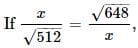find the value of x.

Detailed Solution for Test: Square Root And Cube Root- 1 - Question 1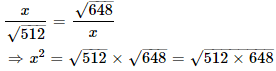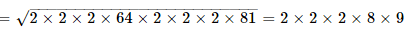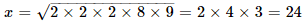Test: Square Root And Cube Root- 1 - Question 2

###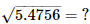Detailed Solution for Test: Square Root And Cube Root- 1 - Question 2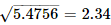Test: Square Root And Cube Root- 1 - Question 3

###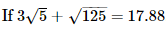then what will be the value of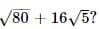Detailed Solution for Test: Square Root And Cube Root- 1 - Question 3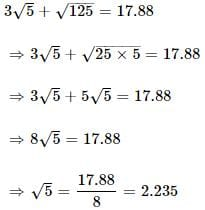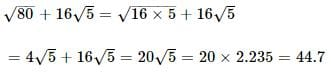Test: Square Root And Cube Root- 1 - Question 4

The cube root of 0.000729 is

Detailed Solution for Test: Square Root And Cube Root- 1 - Question 4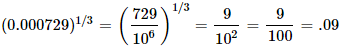Test: Square Root And Cube Root- 1 - Question 5

What is the least perfect square which is divisible by each of 21, 36 and 66?

Detailed Solution for Test: Square Root And Cube Root- 1 - Question 5

LCM of 21, 36, 66 = 2772
i.e., all multiples of 2772 are divisible by 21, 36 and 66
Prime factorization of 2772 is,
2772 = 2 × 2 × 3 × 3 × 7 × 11
i.e., to make it a perfect square, we have to multiply it by 7 and 11
Hence, required number = 2772 × 7 × 11 = 213444

Test: Square Root And Cube Root- 1 - Question 6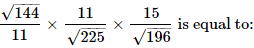Detailed Solution for Test: Square Root And Cube Root- 1 - Question 6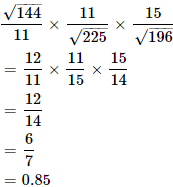Test: Square Root And Cube Root- 1 - Question 7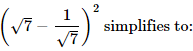Detailed Solution for Test: Square Root And Cube Root- 1 - Question 7

The correct option is Option C.

(√7 - 1/√7)²

= (√7)² - 2 × √7 × 1/√7 + (1/√7)²

= 7 - 2 + 1/7

= 5 + 1/7

= 36/7

Test: Square Root And Cube Root- 1 - Question 8

The square root of 16641 is

Detailed Solution for Test: Square Root And Cube Root- 1 - Question 8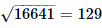Test: Square Root And Cube Root- 1 - Question 9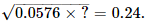Detailed Solution for Test: Square Root And Cube Root- 1 - Question 9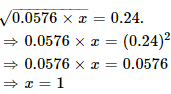Test: Square Root And Cube Root- 1 - Question 10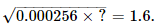Detailed Solution for Test: Square Root And Cube Root- 1 - Question 10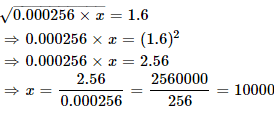## UPSC Prelims Paper 2 CSAT - Quant, Verbal & Decision Making

67 videos|50 docs|151 tests
 Use Code STAYHOME200 and get INR 200 additional OFF Use Coupon Code
Information about Test: Square Root And Cube Root- 1 Page
In this test you can find the Exam questions for Test: Square Root And Cube Root- 1 solved & explained in the simplest way possible. Besides giving Questions and answers for Test: Square Root And Cube Root- 1, EduRev gives you an ample number of Online tests for practice

## UPSC Prelims Paper 2 CSAT - Quant, Verbal & Decision Making

67 videos|50 docs|151 tests